# BUAA-OO-第一单元总结

## 第一次作业分析

### 预处理

• 去除空白符
• 替换连续的 + - 符号以化简处理
• 去除 * 以及 ( 后对表达式不造成影响的 +
• 将乘方符号 ** 替换成 ^ ，便于词法解析的处理
• 将 * 后的 - 替换为 ～ ，便于词法解析处理带符号整数

public static String init(String input) {
String first = input.replaceAll("[ \t]", "");
//remove Blank character
String second = first.replaceAll("\\+\\+|--", "+").replaceAll("\\+-|-\\+", "-");
String third = second.replaceAll("\\+\\+|--", "+").replaceAll("\\+-|-\\+", "-");
//remove continuous "+""-"
String fourth = third.replaceAll("\\*\\+", "*").replaceAll("\\(\\+", "(");
//remove extra "+" behind "^","*","("
String fifth = fourth.replaceAll("\\*-", "*~");
//replace "*-" with "*~"
String expr = fifth.replaceAll("\\*\\*", "^");
//replace "**" with "^"
if (expr.charAt(0) == '+') {
expr = expr.substring(1);
}
return expr;
}


### 代码UML类图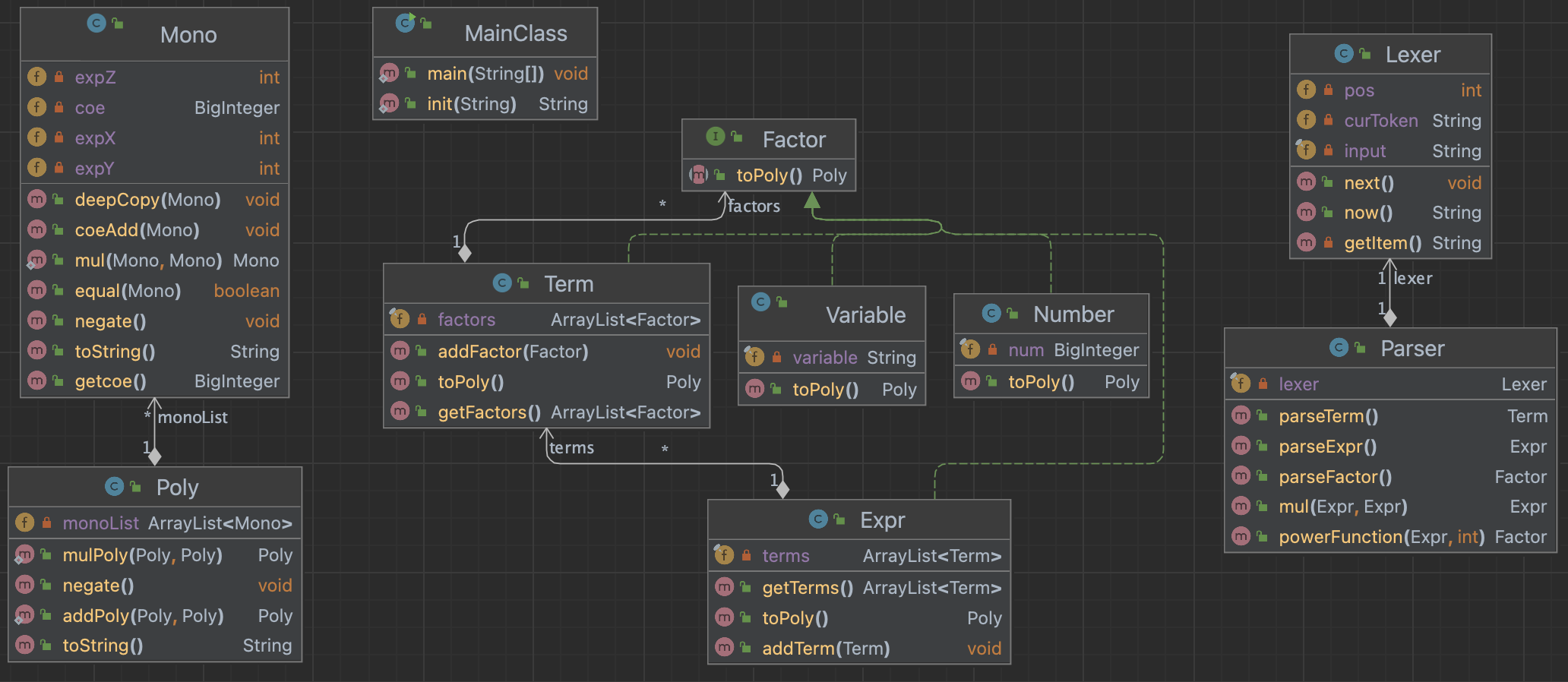### 代码架构分析

#### 表达式解析：递归下降算法

Lexer主要是将表达式分解成一系列基本语法单元，而Parser主要是根据表达式的形式化定义，依靠Lexer分解出的语法单元，递归的生成表达式、项和因子。Paser语法解析中分为如下三个层次递归下降解析：

public Expr parseExpr() {
Expr expr = new Expr();
if (lexer.now().equals("-")) {
lexer.next();
Number neg = new Number(BigInteger.valueOf(-1));
Term term = parseTerm();
} else {
} // Expr begin
while (lexer.now().equals("+") || lexer.now().equals("-")) {
if (lexer.now().equals("-")) {
lexer.next();
Number neg = new Number(BigInteger.valueOf(-1));
Term term = parseTerm();
} else {
lexer.next();
}
}
return expr;
}

public Term parseTerm() {...}
public Factor parseFactor() {...}

• 对于表达式中的 - 符号，直接处理为-1乘以此项
• 对于幂函数，在解析因子时循环乘入项内

#### 表达式展开

• Mono类含有四个成员变量——coe、expX、expY、expZ，分别代表系数和xyz的指数。然后还有toString()方法，将Mono转化成 “coe $\times$ $x^X$ $\times$ $y^Y$ $\times$ $z^Z$” 这种形式。

==Expr.toPoly()==

public Poly toPoly() {
Poly result = new Poly();
for (Term item : terms) {
result = temp;
}
return result;
}


#### 注意事项

==深克隆== 因为运算过程中涉及对象的不同层次间依赖和适用，需采用深克隆！！

### 基于度量的程序结构分析

• 代码规模分析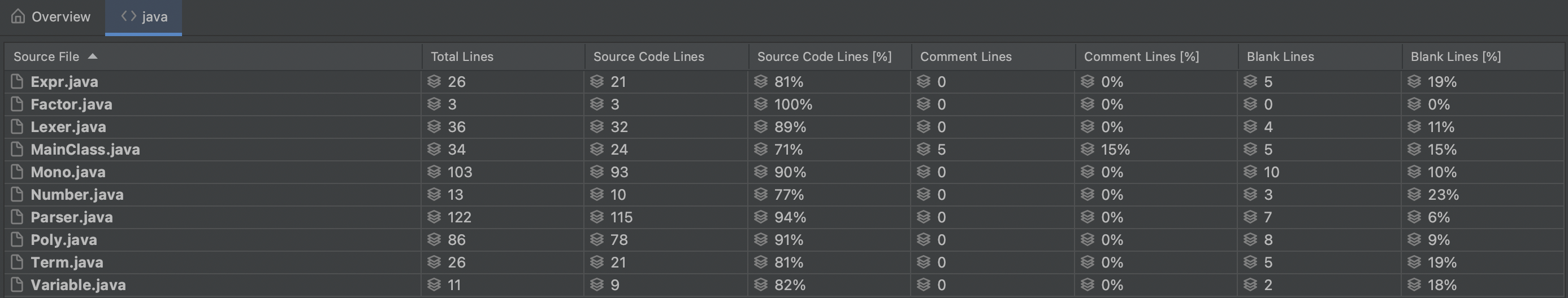• 类复杂度分析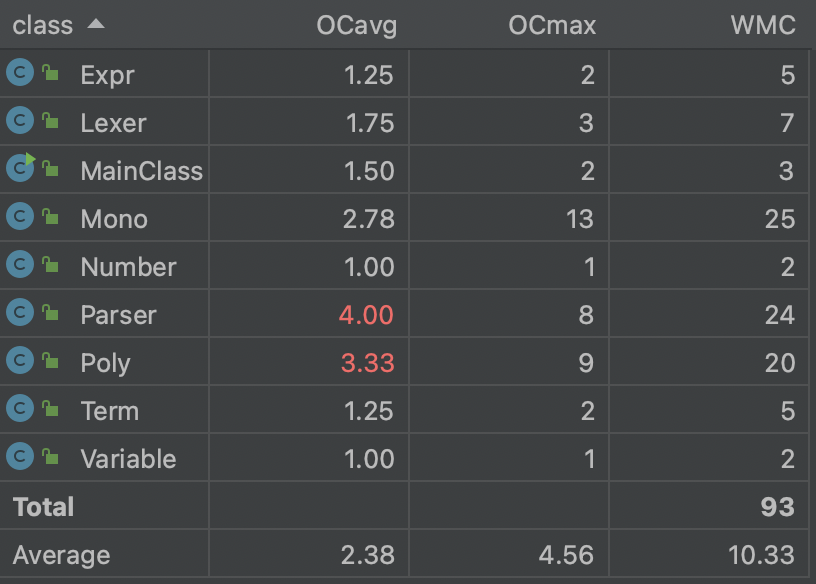• 方法复杂度分析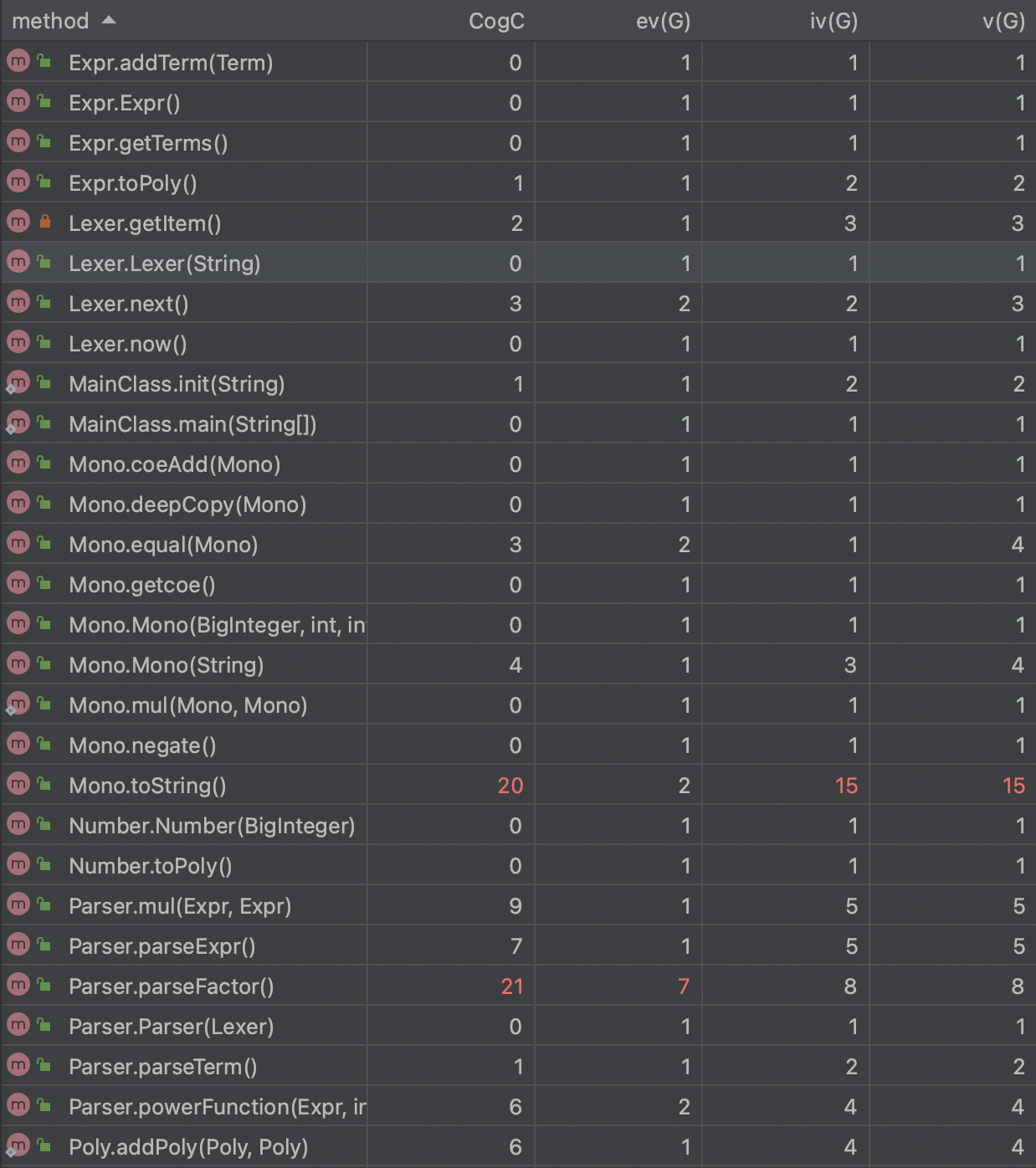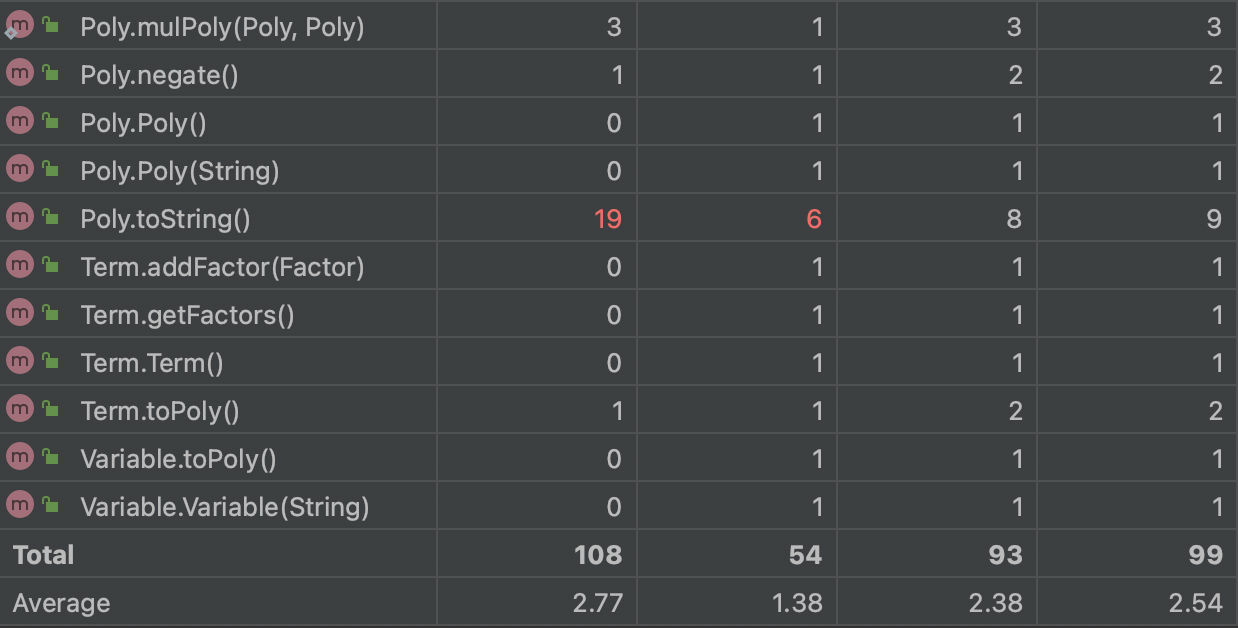• ==ev(G)==：基本复杂度是用来衡量程序非结构化程度的，非结构成分降低了程序的质量，增加了代码的维护难度，使程序难于理解。
• ==iv(G)==：模块设计复杂度是用来衡量模块判定结构，即模块和其他模块的调用关系。软件模块设计复杂度高意味模块耦合度高，这将导致模块难于隔离、维护和复用。
• ==v(G)==：是用来衡量一个模块判定结构的复杂程度，数量上表现为独立路径的条数，即合理的预防错误所需测试的最少路径条数，圈复杂度大说明程序代码可能质量低且难于测试和维护，经验表明，程序的可能错误和高的圈复杂度有着很大关系。

## 第二次作业分析

### 代码UML类图### 代码架构分析

#### 自定义函数

• 存储自定义函数
private static final HashMap<String, String> FUNCMAP = new HashMap<>();
// 函数名与函数体

private static final HashMap<String, ArrayList<String>> PARAMETERMAP = new HashMap<>();
// 函数名和参数

• 套多层循环的方式处理嵌套调用的自定义函数
while (funcBody.indexOf('f') != -1 || funcBody.indexOf('g') != -1
|| funcBody.indexOf('h') != -1) {
funcBody = funcReplace(funcBody);
}


### 基于度量的程序结构分析

• 代码规模分析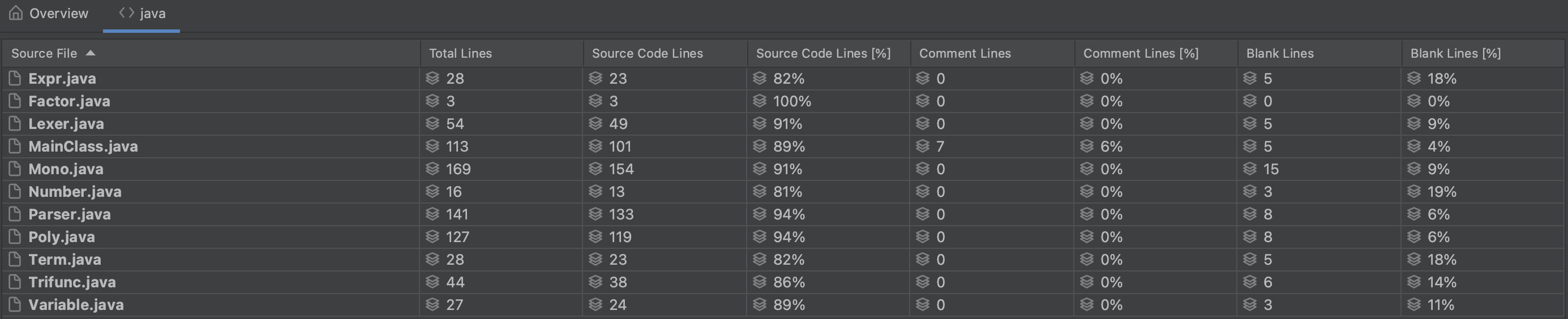• 类复杂度分析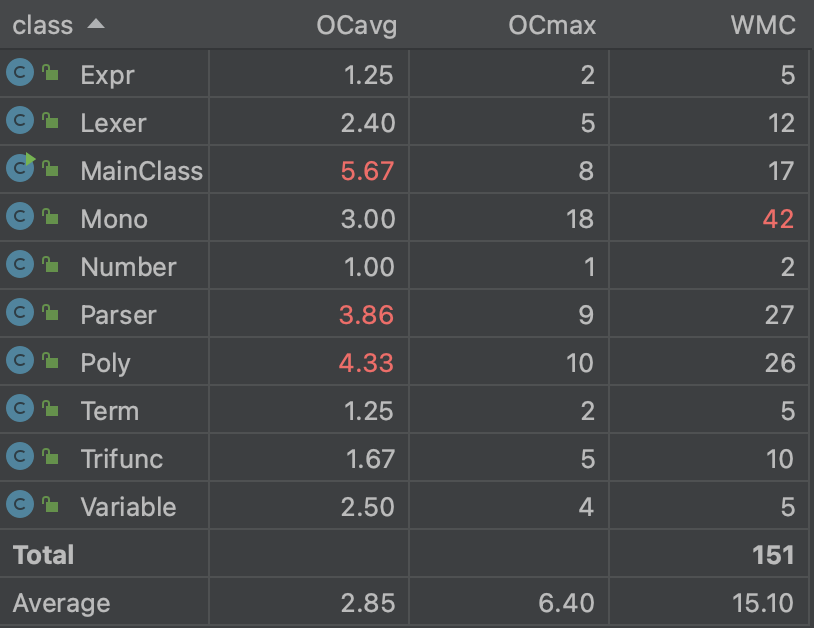• 方法复杂度分析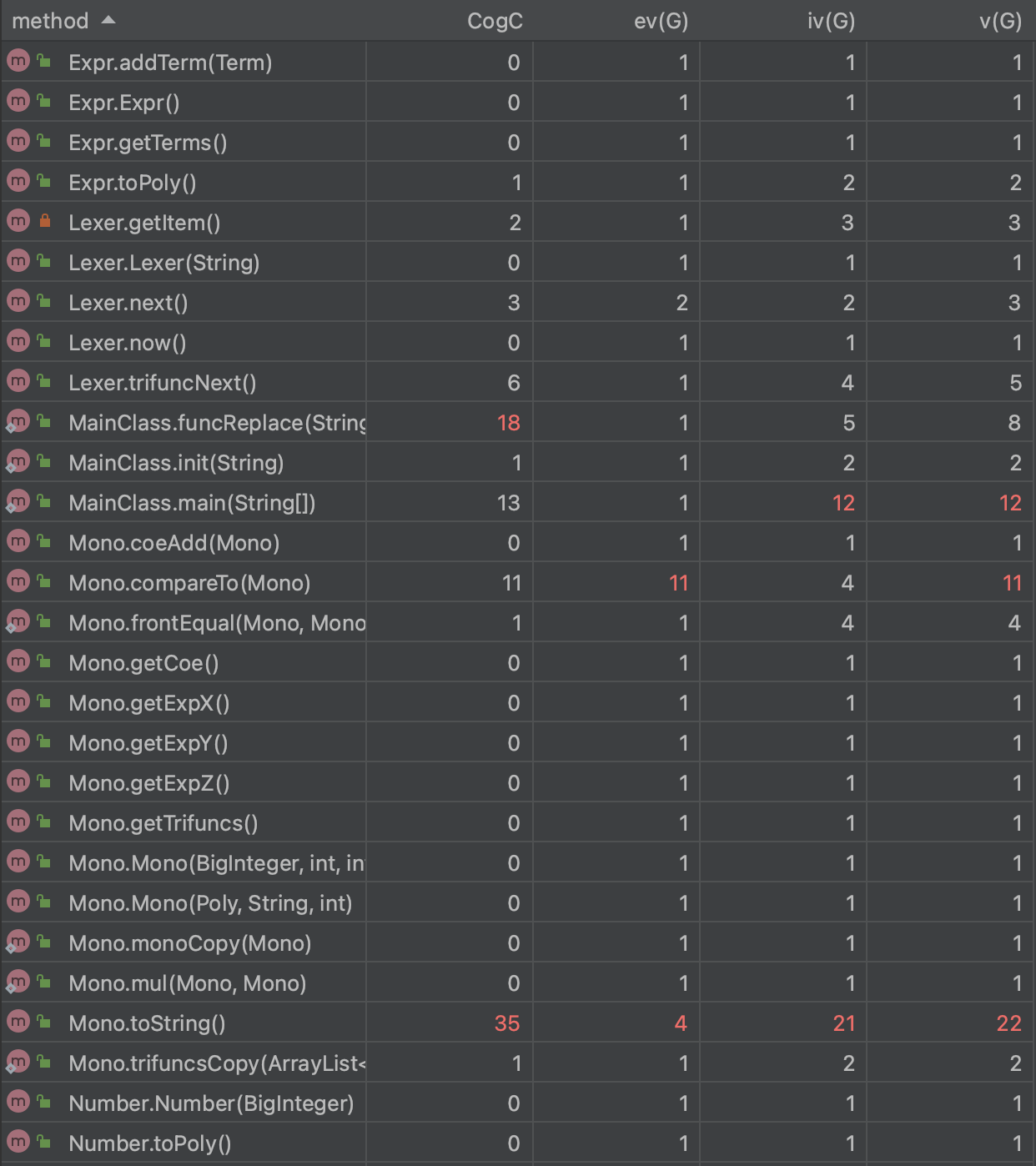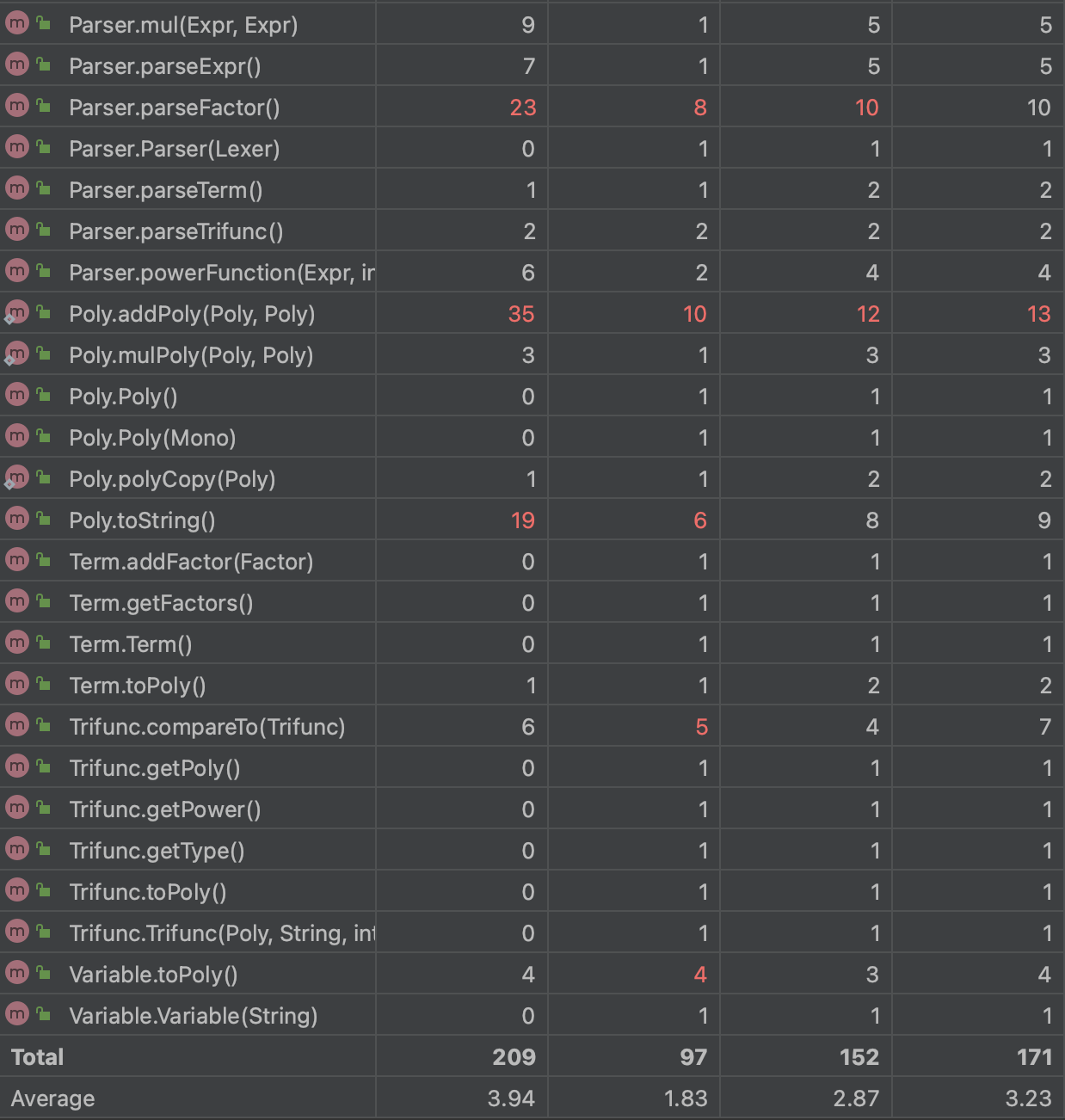• 由于将自定义函数全部放在MainClass中进行处理，此类的复杂度明显超标，对于整体架构不是很友好

## 第三次作业分析

### 代码UML类图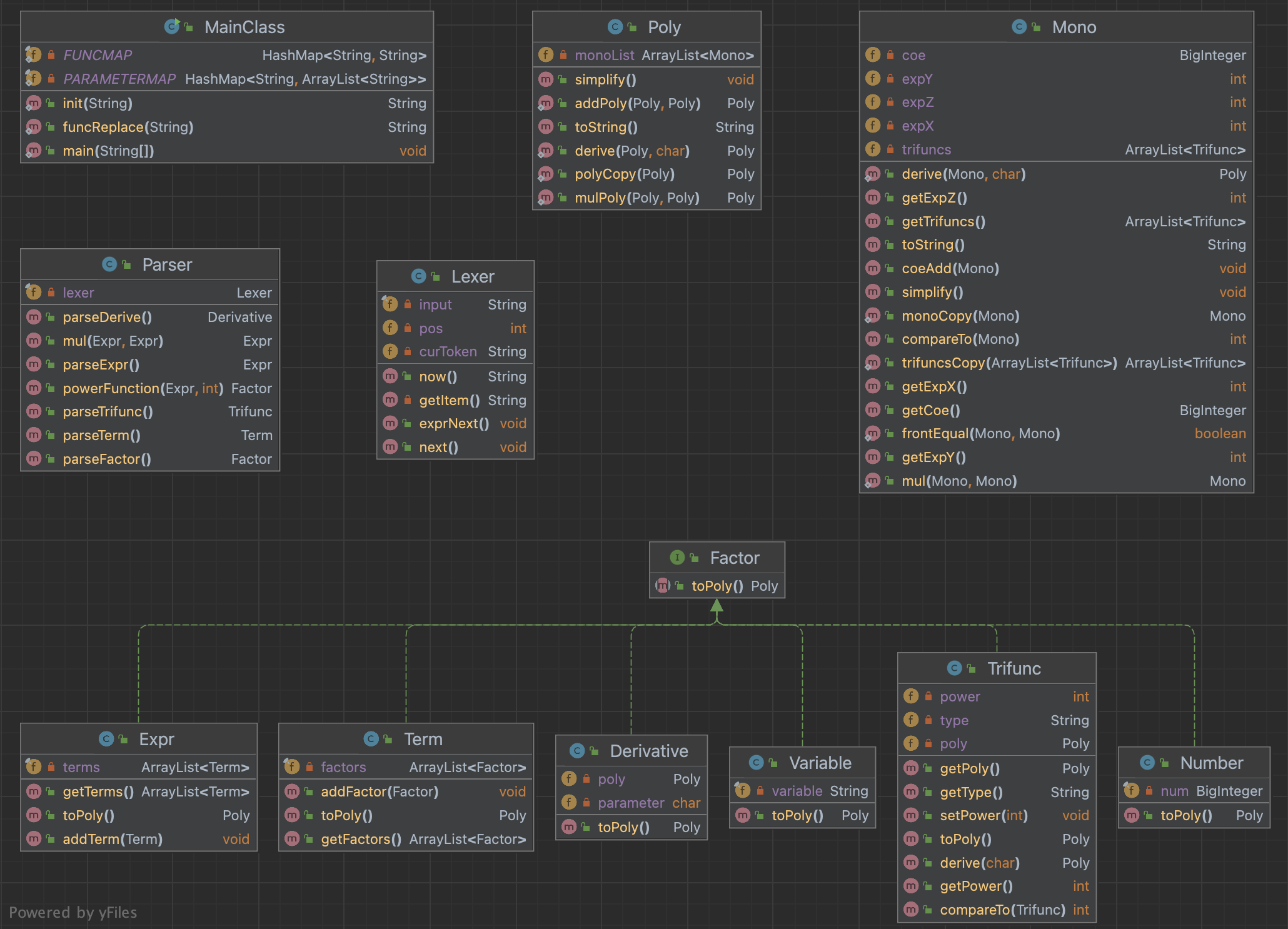### 代码架构分析

• Poly中实现多项式相加的求导
public static Poly derive(Poly poly, char parameter) {
Poly polyResult = new Poly();
for (int i = 0; i < poly.monoList.size(); i++) {
}
return polyResult;
}

• 具体的链式法则与乘法法则在Mono中对单项式的求导实现

### 基于度量的程序结构分析

• 代码规模分析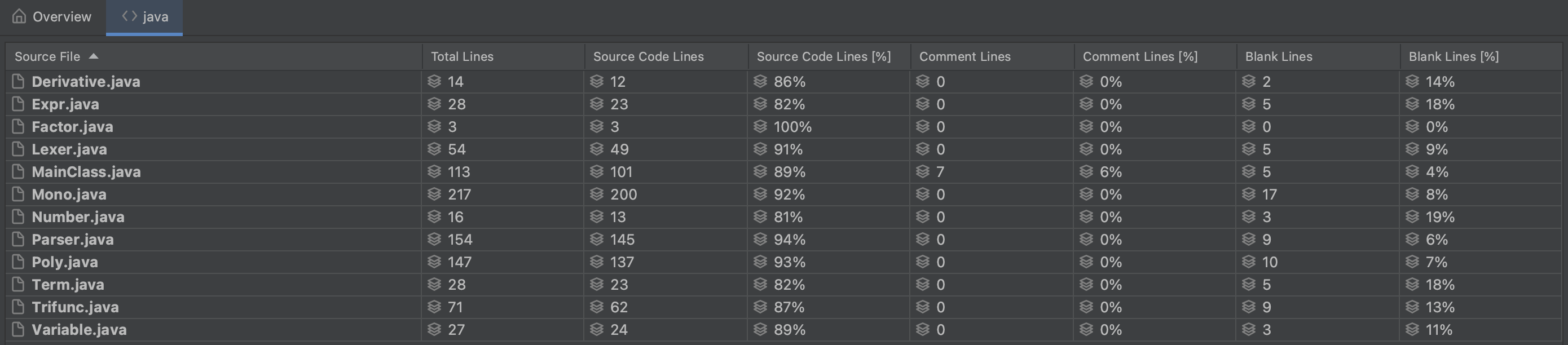• 类复杂度分析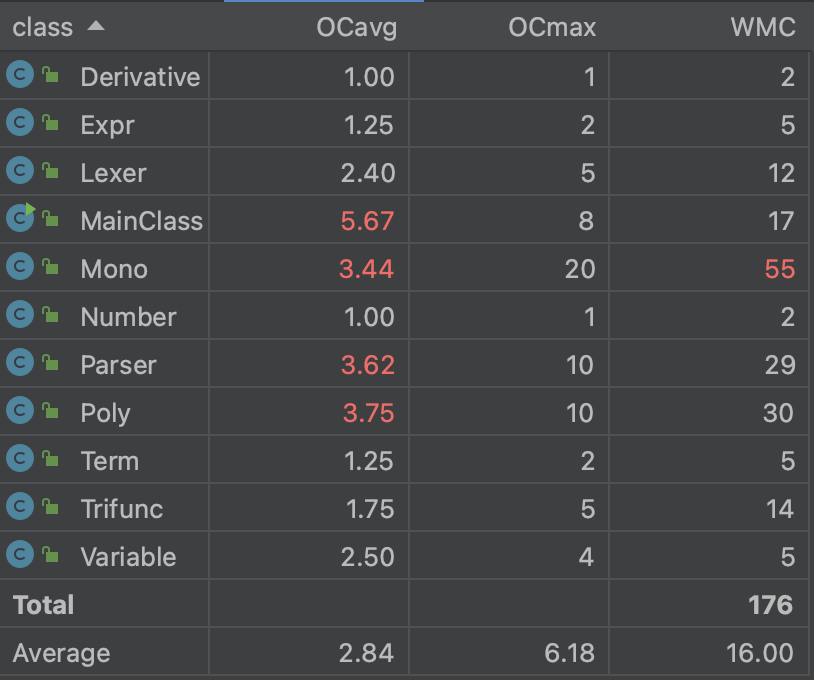• 方法复杂度分析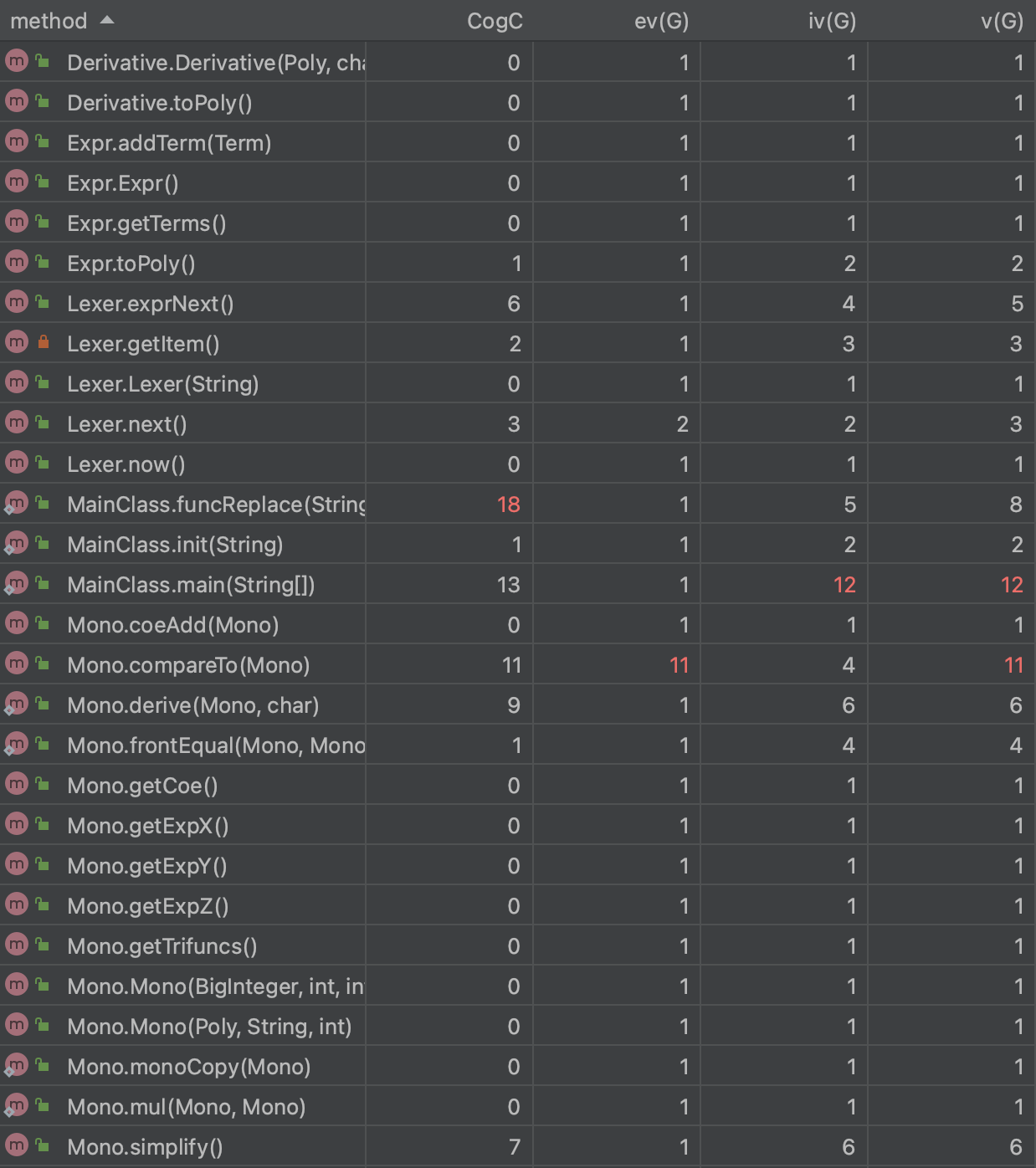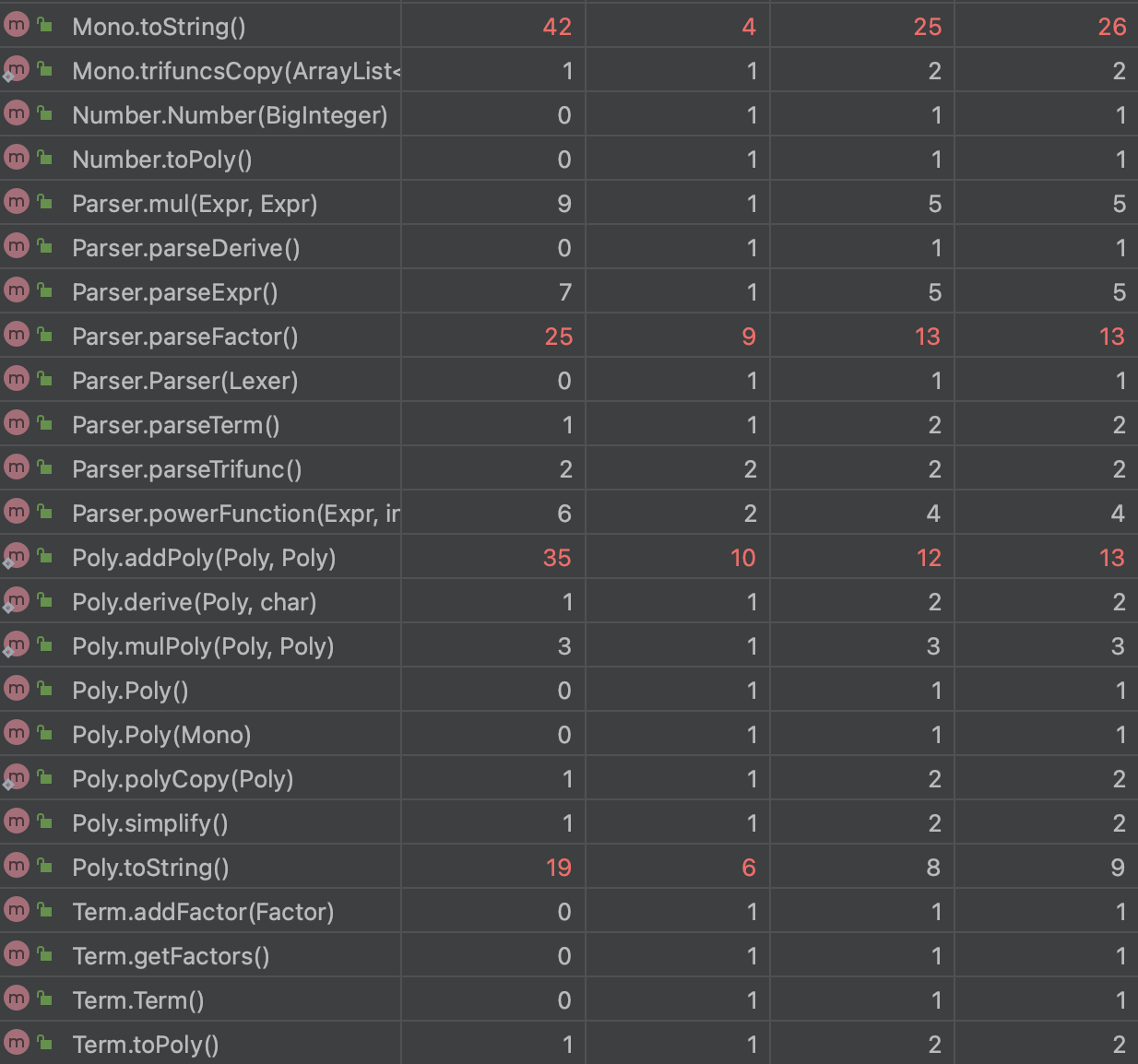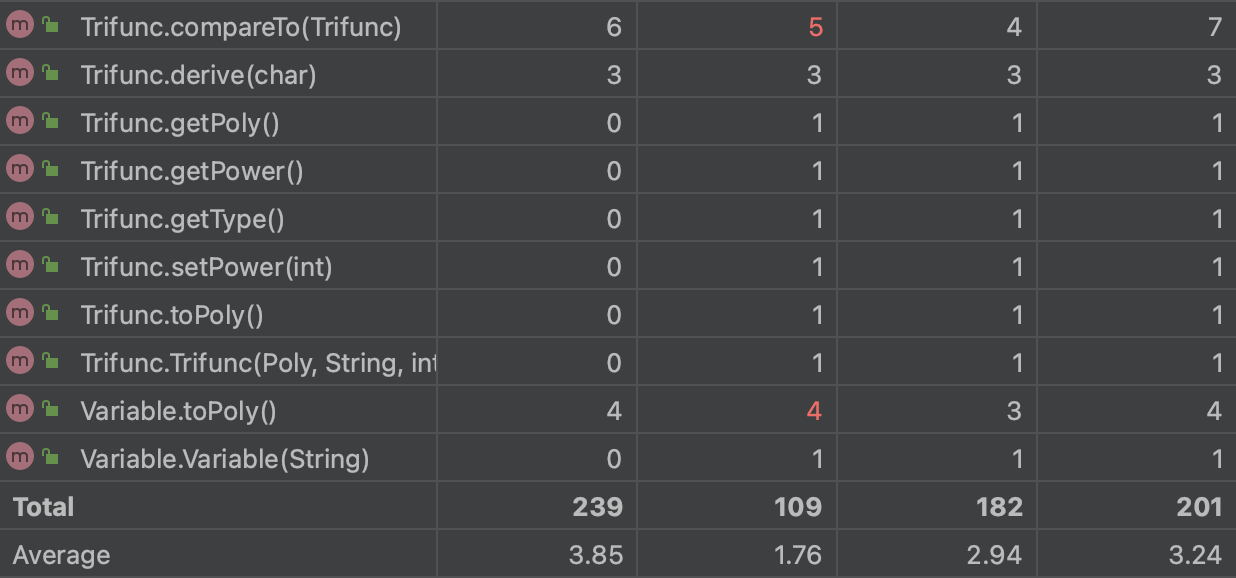## 心得体会

...全文
28 回复 打赏 收藏 举报2023Spring BUAA-OO-Unit1
2023春北航面向对象第一单元BUAA-OO-Unit1 表达式展开
BUAA 面向对象设计与构造 第一单元作业反思与总结BUAA-OO-Unit１